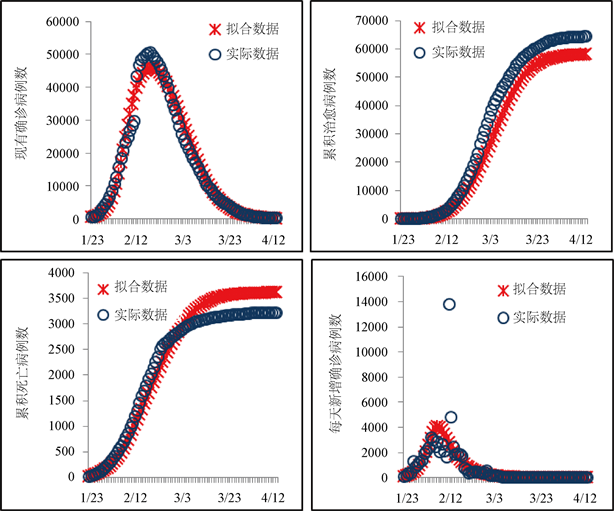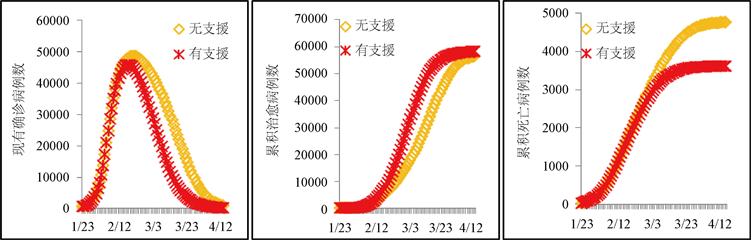# 援助医疗对公共卫生事件的控制及模型构建The Control Effect of Medical Assistance on Public Health Emergencies and Model Construction

DOI: 10.12677/AAM.2021.101002, PDF, HTML, XML, 下载: 18  浏览: 69  科研立项经费支持

Abstract: Based on SEIR infectious disease model, this study constructs a dynamic model that can evaluate the impact of medical assistance on public health emergencies. Utilizing the epidemic data of Hubei province and the data of medical teams that aid in Hubei, this study estimates the parameters of the dynamic model via heuristic algorithm. This study conducts simulation experiments and analyzes the effect of medical assistance on epidemic control by using MATLAB 2018b software. Results show that the medical assistance is able to reduce number of confirmed cases and cumulative number of deaths cases as well as promote the cumulating of cured cases during the epidemic development. The established model of this study reveals the specific role of medical assistance in the public health emergencies.

1. 引言

2019年12月，新型冠状病毒肺炎疫情在湖北武汉爆发，并在两个月的时间内迅速扩散。为此，国家和政府采取了隔离和封城等有效措施控制疫情的发展，同时对新型冠状病毒感染患者进行集中管理和分层治疗。我国的疫情在2020年3月初开始逐步得到了有效地控制，全国累计确诊病例数逐步趋于平缓，全国新增病例数显著减少。在我国疫情常态化管理的同时，仍需要思考的问题是，哪些因素是帮助我国控制新型冠状病毒肺炎疫情的关键因素，如果缺少这些因素会对疫情的发展起到怎样的影响。针对这些问题的思考可以为可能出现的突发公共卫生事件提供防控的建议和策略，最大限度地挽救生命和财产的损失，具有重要的现实意义。虽然以往学者对此进行了诸多的研究，并取得了丰富的研究成果   。比如在控制一些具有传染性的流行疾病时，很多研究都认为对隔离人群的筛查、对确诊人群的治疗和针对密切接触者的追踪能够有效地控制传染病疫情的发展  ；还有一些学者指出人口密度越大和人口流动性越高等城市因素会使得传染病这种公共卫生事件恶化，所以居家隔离和封城也是控制这种类型公共卫生事件的重要手段  ；除此之外，疫苗的提早研发、公共卫生知识的教育、对公共区域的及时消毒和基层群众性自治组织行为都是抑制传染病疫情的措施   。然而，以往针对流行性传染病这种公共卫生事件的防控研究中，却鲜有学者分析医护人员对确诊人群的治疗在控制流行性传染病中的作用并建立相应的数学模型。尤其是在本次的疫情防控中，自2020年1月23日起，全国各省响应国家号召纷纷组建医疗队，前后共有4万多名医护人员奔赴湖北，参与普通感染者和重症感染者的治疗工作，极大地缓解了湖北当地医护人员紧张的局面。既然援助医疗队在流行性传染病这种公共卫生事件中的防控中具有重要的作用，同时以往的理论模型还忽略了医护人员的因素。为此，本研究将在SEIR传染病模型的基础上，建立能够揭示医护人员数量对传染病疫情控制影响的数学模型，并利用湖北省疫情发展状况的数据以及全国各省援助湖北的医护人员数量估计相关的参数。

2. 资料与方法

2.1. 数据来源

2.2. 模型构建

$\frac{\text{d}S}{\text{d}t}=\frac{\text{d}A}{\text{d}t}-\frac{\beta \left(t\right)S\left(t\right)}{N\left(t\right)}\left(I\left(t\right)+\theta E\left( t \right)\right)$

$\beta \left(t\right)=\frac{{\beta }_{0}}{1+{\beta }_{1}{\text{e}}^{ct}}$

$\frac{\text{d}E}{\text{d}t}=\frac{\beta \left(t\right)S\left(t\right)}{N\left(t\right)}\left(I\left(t\right)+\theta E\left(t\right)\right)-\sigma E\left( t \right)$

$\frac{\text{d}I}{\text{d}t}=\sigma E\left(t\right)-r\left(t\right)I\left(t\right)-dI\left( t \right)$

$\frac{\text{d}R}{\text{d}t}=r\left(t\right)I\left( t \right)$

$\frac{\text{d}D}{\text{d}t}=d\left(t\right)I\left( t \right)$

$r\left(t\right)=\frac{k}{1+\epsilon {\text{e}}^{-M\left( t \right)}}$

$M\left(t\right)=\frac{\alpha }{1+\eta {\text{e}}^{-\lambda t}}\left(ts+A\left(t\right)\right)\left(1-{\beta }_{M}\right)$

$r\left(t\right)=\frac{k}{1+\epsilon {\text{e}}^{-\frac{\alpha }{1+\eta {\text{e}}^{-\lambda t}}\left(ts+A\left(t\right)\right)\left(1-{\beta }_{M}\right)}}$

$d\left(t\right)=\phi +\frac{p}{{\text{e}}^{\omega t}}$

2.3. 参数估计及模型评价

Table 1. The meaning and estimates of parameters and variables in the modelFigure 1. The fitting results of the number of confirmed cases, the cumulating of cured cases, cumulative number of deaths cases and number of new confirmed cases every day in Hubei province. The red asterisk represents the fitting result, and the blue circle represents the actual data

2.4. 参数估计及模型评价Figure 2. The comparison of the number of confirmed cases, the cumulating of cured cases, cumulative number of deaths cases, with and without the presence of an assisted medical team. The red asterisk represents the simulated data for the supported group, and the yellow diamond represents the simulated data for the unsupported group

3. 结论

NOTES

*通讯作者。

  Tian, H., Liu, Y., Li, Y., et al. (2020) An Investigation of Transmission Control Measures during the First 50 Days of the COVID-19 Epidemic in China. Science, 1126, 638-642. https://doi.org/10.1126/science.abb6105  白宁, 宋晨玮, 徐瑞. 基于动力学模型的COVID-19疫情预测与控制策略研究[J/OL]. 应用数学学报. http://kns.cnki.net/kcms/detail/11.2040.O1.20200601.0958.002.html, 2020-06-02.  曹盛力, 冯沛华, 时朋朋. 修正SEIR传染病动力学模型应用于湖北省2019冠状病毒病(COVID-19)疫情预测和评估[J]. 浙江大学学报(医学版), 2020, 49(2): 178-184.  漆翠芳, 杨力仁, 杨子轩, 等. 影响新型冠状病毒肺炎省际传播与发展的因素: 基于30个省市的数据分析[J/OL]. 西安交通大学学报(医学版), 2020. http://kns.cnki.net/kcms/detail/61.1399.r.20200417.1413.002.html, 2020-04-21.  李欣, 周林, 贾涛. 城市因素对COVID-19疫情的影响——以武汉市为例[J/OL]. 武汉大学学报(信息科学版), 2020. http://kns.cnki.net/kcms/detail/42.1676.tn.20200514.1542.002.html, 2020-05-15.  倪莉红, Joseph Wu. 动力模型在评价流感疫苗控制大流行疫情效果中的研究[J]. 现代预防医学, 2011(21): 4487-4489.  范如国, 王奕博, 罗明, 等. 基于SEIR的新型肺炎传播模型及拐点预测分析[J/OL]. 电子科技大学学报. http://kns.cnki.net/kcms/detail/51.1207.T.20200221.1041.002.html, 2020-02-22.  詹振运, 张朝霞. 基层群众性自治组织应急行为的合法性检视——以突发公共卫生事件为例[J]. 河北科技大学学报(社会科学版), 2020, 20(20): 104-112.  中华人民共和国国家卫生健康委员会. 新型冠状病毒感染的肺炎疫情最新情况[EB/OL]. http://www.nhc.gov.cn  湖北省卫生健康委员会. 湖北省新型冠状病毒感染的肺炎疫情情况通知公告[EB/OL]. http://wjw.hubei.gov.cn  湖北省统计局. 2019年统计年鉴[EB/OL]. http://tjj.hubei.gov.cn/tjsj/sjkscx/tjnj/qstjnj  Anderson, R.M., Anderson, B. and May, R.M. (1992) Infectious Diseases of Humans: Dynamics and Control. Oxford University Press, Oxford.  湖北省卫生健康委员会. (健康报)湖北: 超17万医务人员上一线[EB/OL]. http://wjw.hubei.gov.cn/bmdt/ztzl/fkxxgzbdgrfyyq/fkdt/202002/t20200201_2017410.shtml, 2020-02-01.  Armour, G.C. and Buffa, E.S. (1963) A Heuristic Algorithm and Simulation Approach to Relative Location of Facilities. Management Science, 9, 294-309. https://doi.org/10.1287/mnsc.9.2.294